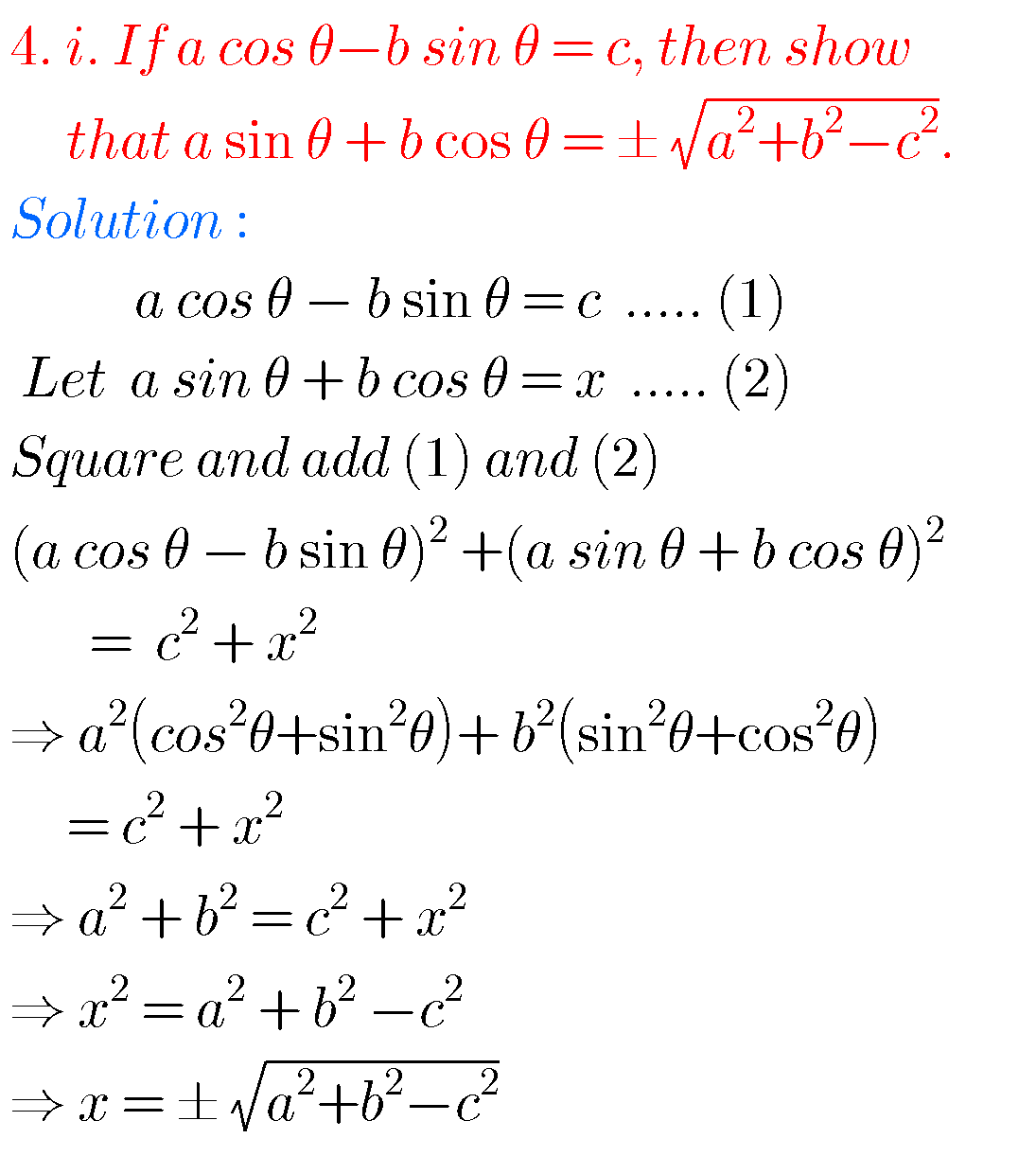## 1st Inter exercise 6(a) Solutions maths 1A ,chapter 6 Trigonometric Ratios upto Transformations

Inter maths 1A chapter 6 exercise 6(a) solutions Intermediate mathematics 1st year chapter 6 Trigonometric Ratios upto Transformations textbook solutions for exercise 6(a). These solutions are very easy to understand. Study the text boo lesson very well. Observe the example problems and solutions in the text book. Try them. Observe the given solutions and try …